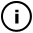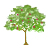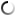# Sunday, April 04, 2010

## Molten pixels

int counter = 0;

void setup(){

size(800,400);
background(255);
noStroke();

initialise();
}

void initialise(){

background(255);
counter = 0;

for (int i = 0; i< width;i++){
fill(int(random(0,255)));
rect(i,0,1,1);
}

}

void draw(){
if (counter == height) {
noLoop();
}

for (int i = 0; i< width; i++){

float averageValue = 0.0;
averageValue = ( red(get(i-1, counter))  +  red(get(i, counter))  +  red(get(i+1, counter))  ) / 765.0 + 0.05;

fill((averageValue - int(averageValue))*255);
rect(i,counter+1,1,1);

}

counter++;
}

void mousePressed(){
initialise();
loop();
}

## infosubmitted by: DARYL_Gamma
views: 151
as seen in the picture in Wolfram's "new kind of science" page 243 http://www.wolframscience.com/nksonline/page-243This sketch has a parent
Tags: ca, cellular, automaton

## commentsloading...